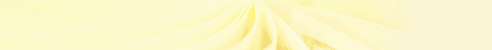Payroll Formula FAQ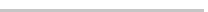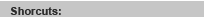Select an Item ----------------------- Dealers Promotion FAQ Training Registration Form Download Program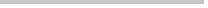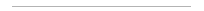Payroll Fomula Frequently Asked Questions

2. What is "OTA"? "OTA" is stand for Over Time Allowance. <<Answer

3. Formula for calculating Daily, Hours, and Monthly employee's basic pay. <<Answer

5. "TOVER_T" is used to calculate the Total Overtime Hours for an employee. <<Answer

6. "GETLEAVE" or “GL” is used to calculate the total leave taken for the month.<<Answer

7. "GETOTAL" is used to calculate the Total Leave Days. <<Answer

8. "GETI" is used to calculate the total leave taken for the current payslip. <<Answer

9."GETV" is used to calculate the total amount of leave by separate code. <<Answer

10. "GETAUDF" is used to obtain the user defined value and description. <<Answer

11. AWKD" is used to calculate the actual working days of employee. <<Answer

12.Bonus Cycle is used to print bonus payslip. <<Answer

13. "FSTWKD" = Fist Half Working Day and "SNDWKD" = Second Half Working Day <<Answer

14. "GETBPAY" is used to calculate basic pay before basic pay adjusted.
"WKDB" is used to calculate the working days before basic pay adjusted.
"GETAPAY" is used to calculate basic pay after basic pay adjusted.
"WKDA" is used to calculate the working days after basic pay adjusted.

15. "GETLEVB" is used to calculate the entitle leave balance. Calculate by entitle - taken.
eg. - GETLEVB("L01)

16. "GETUDESC" used to get the allowance user defined field.

17. "GETENLEV" used to get the entitle leave in reports.
eg. - GETENLEV(mtmptable.employee,"L01")

18. "GETLEUDF" used to get the leave user defined field.
eg. - GETLEUDF("<leave desc>","1")

19. "GETOT"
a) - GETOT(<OT Code>,mtmptable.employee)
used to calculate the sum of overtime amount according to OT code.
b) – GETOT (“”,employee.employee, “L01”)
used for calculate total day that have overtime but on that day the employee got apply leave (except annual leave).

20. "GETOTUDF" used to get the OT user defined field.
eg. - GETOTUDF(mtmptable.otdesc1,"1")

* --- Pre-released version ------------------------------------------------------------------

21.To write formula for basic pay if basic pay adjustment based on date:
- In Admin, Boss Setup, Page 1:
A). Daily Worker - IIF(!EMPTY(GETBPAY).OR.!EMPTY(GETAPAY),ROUND(GETAPAY,2)+ROUND(GETBPAY,2),EMPLOYEE.BASIC*DRE)

B). Hourly Worker - IIF(!EMPTY(GETBPAY).OR.!EMPTY(GETAPAY),ROUND(GETAPAY,2)+ROUND(GETBPAY,2),EMPLOYEE.BASIC*HRE)

C). Monthly Worker - IIF(!EMPTY(GETBPAY).OR.!EMPTY(GETAPAY),ROUND(GETAPAY,2)+ROUND(GETBPAY,2),EMPLOYEE.BASIC)

D). New Worker - IIF(!EMPTY(GETBPAY).OR.!EMPTY(GETAPAY), ROUND(GETAPAY,2)+ROUND(GETBPAY,2), IIF(DAY(EMPLOYEE.JOINED)=1 .AND. EMPLOYEE.PAYBASIC="M",EMPLOYEE.BASIC, IIF(DAY(EMPLOYEE.JOINED)=16 .AND. EMPLOYEE.PAYBASIC="M", EMPLOYEE.BASIC/2,EMPLOYEE.BASIC/WKD*NWKD)))
Note: get half month salary if joined in 2nd half.

* --- Pre-release Admin Setup -------------------------------------------------------------------------------------

22. Calculate Leave by Proportion <<Answer

23. Advance Pay is follows transaction date when update to Accounting.

24. Fields Expression and the Code for Year-To-Date amount in payslip. <<Answer

25. If Bonus is print out in separate payslip, but need to include in month pay year-to-date total. <<Answer

26. Formula for EPF :- <<Answer

27. How to calculate PCB Base for monthly pay ? <<Answer

28. How to calculate Bonus PCB Base ? <<Answer

"WKD" is for calculating total number of working day in that particular month. In order for the "WKD" to work, first, you required to enter the work calendar. Next, you need to key in the calendar code in the "employee". Then the WKD will work. Example
(EMPLOYEE.BASIC+OTA)/1/(WKD*8)*1.5*EEOVER_T.HOURS

Note: "NON" would be included in wkd.<<Go Top

"OTA", calculated the total overtime pay. This is used when some company need to calculate the employees basic includes overtime allowance. Before process to the next step, you need to go to the code defined and select allowance/deduction code and choose which allowance or deduction, which is, need for the OTA. Example above

Example if you select food as allowance and included with OTA is "Y". Then the basic pay is added with the OTA value, when calculating payslip.

This goes same to HRD, LEAVE, EPF, and SOCSO. Except TAX, I'm still working on it.

Note : "DOTA" calculates the Daily Total OTA
"FOTA" calculates the First Half Total OTA
"SOTA" calculates the Second Half Total OTA
* can be used in pre-released version only, used in formula and user-defined. <<Go Top

Before process to enter basic pay. You are required go to the boss setup to see if the calculate basic is entered. If not, you need to enter the code in order for the system to know which employees are daily worker, monthly worker and hourly worker.

Example:
Daily worker basic pay is entered in this way. EMPLOYEE.BASIC+DRE.
Remember DRE is for Daily worker only.

Monthly Worker basic pay is entered in this way. EMPLOYEE.BASIC.

Hourly Worker basic pay is entered in this way. EMPLOYEE.BASIC+HRE.
Remember HRE is only for Hourly worker only. <<Go Top

Code Definition
----------------------------------------------------------------------------------------------
Employee basic pay = EMPLOYEE.BASIC
Enter edit Overtime hours = EEOVER_T.HOURS
Enter edit leave days = EELEAVE.DAYS
Calculated working days = WKD
Calculated Leave = LEV
Calculated Overtime allowance = OTA
Daily Rate Worker pay = DRE
Hourly Rate Worker pay = HRE
Calculated Levy = HRD
Calculate Total Overtime Hours = TOVER_T
----------------------------------------------------------------------------------------------
Example
Enter basic pay in to the formula, employee.basic
Enter Basic pay with overtime allowance into the formula, employee.basic+OTA
Enter Basic pay with Leave allowance into the formula, employee.basic+LEV

Enter basic pay for the hourly rate worker, employee.basic+HRE
Enter basic pay for the daily rate worker, employee.basic+DRE

* ----- Formula only ---------------------------------------------------------------------

"TOVER_T" is used to calculate the total overtime hours for an employee.
If employer wants to give overtime allowance to employee whose basic pay exceeds certain amount, e.g. if a manager's salary exceed \$2000.00, he must work more than 10 hours of overtime, therefore he can get the overtime allowance.
To calculate the overtime amount when the condition is valid, user can change the overtime hours required in the formula in maintenance code - overtime.
For example:
1.5 * ((EMPLOYEE.BASIC+OTA)/(WKD*8)) * iif(tover_t>7,eeover_t.hours,0) <<Go Top

"GETLEAVE" or “GL” is used to calculate the total leave taken for the month.
This is used for maintenance - code setup - formula.
For example
i.GETLEAVE("L01") - For Days
ii.GETLEAVE("L10",1) - For Hours
iii.GETLEAVE("L10","",1) - To calculate how many times of leave taken, but not the actual days/hours
iv.GETLEAVE("L10","","","1ST") - To calculate leave days taken base on cycle
Or
GL2(“L10”, “1ST”) – To calculate leave days taken base on cycle
v. GL(“L01”,””,””,””,0.5) – To calculate total annual leave that the leave taken is over half day leave. <<Go Top

"GETOTAL" is used to calculate the total leave days. Usually, this is used in Allowance formula. For example, if allowance is given if employee does not take any leave in the process month. The formula will be:
iif(GETOTAL() >= 2, 0, 50)

* ----- Payslip Format only --------------------------------------------------------------<<Go Top

"GETI" is used to calculate the total leave taken for the current payslip.
User can add field in payslip to list out the number of leave taken.
For example:
Note: if want to get only total leave with leave code “L01”,
GETI("L01",””,mtmptable.employee,mcycle)
Note: If want to print leave hours, the code change to
GETI("L01",1,mtmptable.employee,mcycle)
Note: If want to sum all leave days exclude PAID leave,
GETI("",”” ,mtmptable.employee,"","Y")
(“Y” means wants to include ONLY leave code that has no formula). <<Go Top

"GETV" is used to calculate the total amount of leave by separate code.
User can add field in payslip :-
GETV("L07",mtmptable.employee,"mtmptable",mcycle)- pre-released version
GETV("L07",mtmptable.employee) - standard version <<Go Top

."GETAUDF" is used to obtain the user defined value and description.
For example:
- to get description
- to get value
** for the 1st parameter, it is the allowance/deduction description
for the 2nd parameter, it is the user defined column value
for the 3rd parameter, it is the allowance/deduction code, if you put “”, then the prg will calculate all allowance/deduction for you
for the 4th parameter, it is either “1” or “2”. “1” means to get the value for all employees, “2” means to get the value for the particular employee code based on the 5th paratemeter.
For the 5th parameter, it is the employee code.

Note : "GETOTUDF" is used to obtain OT user defined field
"GETLEUDF" is used to obtain Leave user defined field

* --- Both formula & payslip format ----------------------------------------------------<<Go Top

"AWKD" is used to calculate the actual working days of employee, i.e. it will count on the code with WKD and HLF. "WKD", "NWKD", "RWKD" and "CWKD" will count on the WKD, HLF, NON and PUB. If user don't want to include non-working day in calculate basic pay of new/resign worker, just leave the day be blank. <<Go Top

Bonus Cycle is used to print bonus payslip.
- If user wants to separate the bonus with month pay, pls put "BON"
- If user wants to combine the bonus with month pay, pls put "PAY"
- If user wants to combine the bonus with 1st half pay, pls put "1ST"
- If user wants to combine the bonus with 2nd half pay, pls put "2ND" . <<Go Top

"FSTWKD" used to calculate first half working day
"SNDWKD" used to calculate second half working day <<Go Top

Calculate Leave by Proportion
- User must enter the entitle leave days of employee for the year.
- E.g. If a employee joined on 1/4, and the entitle leave days for the first year is 12 days.
When enter data in the Entitle Leave Defined, the entitle should be 9.
- If the employee not entitle any leave before he is confirm. Must put "Y" in Admin Setup,
Calculate Entitle Leave not include Probation Employee, and the employee status must be "P". <<Go Top

Get Year-To-Date amount in payslip:
field expression :
GETYTD(<MONTH END CODE>,MTMPTABLE.EMPLOYEE, <TYPE>)
Eg: GETYTD(”BASICPAY”,MTMPTABLE.EMPLOYEE, “BP”)
Month End Code & Type

 Code Description Type BASICPAY Basic Pay BP BONUS Bonus BO EMPEE_EPF Employee EPF E1 EMPER_EPF Employer EPF E2 BONEE_EPF Employee Bonus EPF BE BONER_EPF Employer Bonus EPF BR EMPEE_SOCSO Employee SOCSO S1 EMPER_SOCSO Employer SOCSO S2 EMPEE_PCB Employee PCB P1 EMPER_PCB Employer PCB P2 BONUSPCB Bonus PCB BC LEAVE Leave Amount LE ADVPAY Advance Pay AV HRD_DED HRD Deduction HD GROSSPAY Gross Pay GP NETPAY Net Pay NP ZAKAT Zakat Amount ZA MEDICAL Medical leave MD ANNUAL Annual Leave AL

Note : For Allowance, Deduction and Overtime, Code refer to the code maintenance, and type are: "A" for Allowance, "D" for Deduction and "OT" for Overtime. <<Go Top

If Bonus is print out in separate payslip, but need to include in month pay year-to-date total, the following variables are used:-
(a) mtmptable.bonusamt (Current month bonus)
(b) mtmptable.bonee_epf (Current month bonus epf : employee)
(c) mtmptable.boner_epf (Current month bonus epf : employer) <<Go Top

Formula for EPF :-
(a). Employee : (EMPLOYEE.BASIC+EPFA+EPFOT+EPFLEV)*0.11
OR
Employee : (MEPFVALUE+MBONVALUE)*0.11
Note : To calculate normal pay and bonus EPF.

(b). Employer : (EMPLOYEE.BASIC+EPFA+EPFOT+EPFLEV)*0.12
OR
Employer : (MEPFVALUE+MBONVALUE)*0.12
Note : To calculate normal pay and bonus EPF. <<Go Top

PCB Base = (BasicPay + Allowance - Deduction + OT - Leave) - maximum EPF deduction
Note: Allowance, Deduction, OT and Leave are added ONLY IF it is included in TAX (i.e. Tax is not 0).
<<Go Top

Tick on 'Formula Visible' in the screen of Print Payslip before print/preview payslip. <<Go Top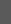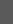>>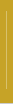>>>>>> Report Writer System >> Emas.net Application